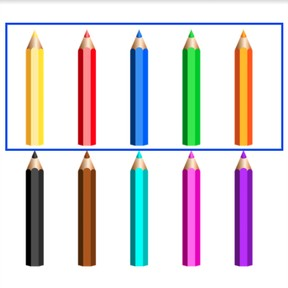Recognizing half in quantities to 10

# Recognizing half in quantities to 10

Recognizing half in quantities to 108,000 schools use Gynzy92,000 teachers use Gynzy1,600,000 students use Gynzy

## General

Students can recognize half quantities fro 0-10. They learn the concept of half by dividing numbers into groups.

K.CC.C

## Relevance

You learn this so you know if you can split something evenly. An example would be if you want to share candies. If each gets half, you have shared them evenly.

## Introduction

Students practice with recognizing more/less to 10 by counting objects in the images and comparing them with each other.

## Development

Explain that half is created when you divide a number into two equal groups. Examples would be cookies or pencils. If two students get to share 4 cookies, they each get 2 cookies. Each student gets half of the cookies. Show that you can determine half of an amount by dividing it into two equal groups- demonstrating with the pencils. Take 8 pencils and divide them between two students. Each student gets half, which is 4 pencils. Show that you can also have "half" of something- use for example a sheet of paper or other object which can be halved. Check that students understand the idea of half by asking them to compare the raspberries.

Check that students understood half by asking the following questions:
What does half mean?
Can you divide something in the class into two groups?
When is it useful to know what half of something is?

## Guided Practice

Students practice by comparing different images with each other and indicating when half of something is in a different color.

## Closing

Check that students understood by asking them to divide the blocks of cheese among the mice so each mouse gets half. Discuss that it is useful to divide evenly because that way you are sure everyone has the same amount.

## Teaching Tip

Students who have difficulty can be supported by the use of blocks. Ask them to take an equal amount of blocks and divide the number into two groups using the blocks. Student looking to be challenged can be encouraged to already practice with half of numbers to 20.

## Instruction materials

Pencils, blocks or other material that you can divide into groups.

### The online teaching platform for interactive whiteboards and displays in schools

• Save time building lessons

• Manage the classroom more efficiently

• Increase student engagement